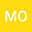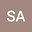Exponential stability of linear systems under a class of Desch-Schappacher perturbations.
••Safae El Alaoui
Universite Sidi Mohamed Ben Abdellah de Fes Ecole Normale Superieure de Fes
Author Profile## Abstract

In this paper we investigate the uniform exponential stability of the system $\frac{dx(t)}{dt}=Ax(t)-\rho Bx(t), \; (\rho >0),$ where the unbounded operator $A$ is the infinitesimal generator of a linear $C_0-$semigroup of contractions $S(t)$ in a Hilbert space $X$ and $B$ is a Desch-Schappacher operator. Then we give sufficient conditions for exponential stability of the above system. The obtained stability result is then applied to show the uniform exponential stabilization of bilinear partial differential equations.
21 May 2022Submitted to Mathematical Methods in the Applied Sciences
23 May 2022Submission Checks Completed
23 May 2022Assigned to Editor
02 Jun 2022Reviewer(s) Assigned
11 Oct 2022Review(s) Completed, Editorial Evaluation Pending
18 Oct 2022Editorial Decision: Revise Major